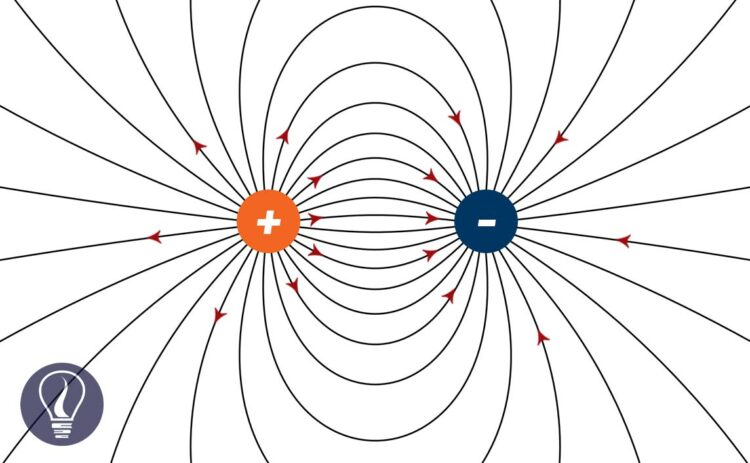More results...

Generic selectors
Exact matches only
Search in title
Search in content
Post Type Selectors

# Electric Field LinesB.Sc (Hons, USJ) (Polymer Science and Technology, Chemistry, Physics)
Categories
Last Updated On : September 22, 2023
Published Date : September 22, 2023

When a point light positive charge is placed in a certain area, the path it follows due to electric force is called an electric field line. Electric field lines spread from positive charges.

If there is a high density of electric field lines at a point, ( if a higher number of field lines pass through that point) the electric field intensity of that point is high. If no lines of electric field lines pass through a point, the electric field intensity at that point is zero. (figure 02)

## Properties of electric field lines

1. Electric field lines never intersect each other
2. Electric field-lines may start from positive charges and end at negative charges
3. Electric field-lines may start from positive charges and end at infinity
4. Electric field lines may start from infinity and end at negative charges
5. If there are no field lines at a point, the electric field intensity of that point is zero. That point is known as the 'Neutral point' (Figure 02).
6. There is always repulsion between field lines
7. Electric field-lines are perpendicular to the charged surface

## Electric flux

Electric flux is the number of electric field lines across a unit area. The electric flux that passes perpendicular to a unit area, is the electric field intensity of that area.

Where,

• φ  = Electric flux
• E = Electric field intensity
• A = Surface area

If the field lines are not perpendicular to the surface, the electric flux can be calculated as follows.

Where θ is the angle between the surface and the field lines.## References and Attributes

### Figures:

The cover image was designed using an image by Geek3, licensed under CC BY-SA 3.0, via Wikimedia Commons

Figure 01: Contains an image by Sharayanan, licensed under CC BY-SA 3.0, via Wikimedia Commons

Figure 01: Contains an image by Nein Arimasen, licensed under CC BY-SA 3.0, via Wikimedia Commons

Figure 02: Contains an image by Geek3, licensed under CC BY-SA 4.0, via Wikimedia Commons